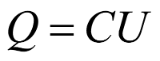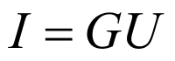# 2. Calculation of grounding resistance is a calculation of the electric field

From the series of articles "Grounding resistance calculation is almost simple".

Anyone familiar with the calculations of electrostatic fields knows that the medium there is characterized by permittivity, the source of the field is an electric charge, and the main parameter is the potential created by the totality of electric charges. The field of direct electric current is completely equivalent to electrostatic one. Only in this field the medium (soil) is characterized by resistivity, instead of a charge an electric current appears in the soil, and the potential remains the potential. In the electrostatic field, the connection between the charge Q and the potential U is determined by the electric capacitance C(3)

In the field of electric current, the connection between current and potential determines the conductivity(4)

If the potential U from the current I is known, the conductivity of the grounding device, and therefore the ground resistance, is determined elementarily by the formula

Rg = 1/G

(5)